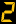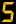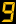# Atomic Orbit Simulation

This simulation employs the use of equation 7 to generate a suite of stable orbits that may be occupied by a quantity of electrons to form an atom. The "constant", a, varies with the number of protons, as one might expect, and knowing three characteristics of a an exponential function is used. The three characteristics of a are:

1) when no proton is present, a must be zero;

2) when one proton is present, a must be equal to the fine structure constant;

3) when a large number of protons is present, 2a asymptotically approaches one.

Thus, a = (1 - exp(K * p)) / 2 where: K = -0.0147022555 and p = the number of protons representing charge in the nucleus.

The measured values for the atomic radii are courtesy of www.webelements.com.

Particle sizes are not to scale.